# big numbers

Numbers go on for ever, but our notation does not. If you want to write down the value of a very big number (way bigger even than a googolplex ), the mathematical notations for factorial or exponentiation, the fastest growing 'conventional' functions, eventually becomes unweildy; you get too many factorials or exponents to manipulate easily. Something more extensible is needed.

The so-called "large primes " -- useful for cryptography -- are relatively small compared to the kind of numbers that soon arise in these notations.

## Knuth's up-arrow notation

In 1976 Donald Knuth published his up-arrow notation for large numbers. The arrows 'associate to the right' (following exponentiation). So a ^ b ^ c ^ d means a ^( b ^( c ^ d )), etc. This association gives the largest value.

1. m n = m + m + ... + m ( n terms) = m × n
2. m ^ n = m m ... m ( n terms) = m n
• m ^1 = m
• m ^ n = m ( m ... m ( n -1 terms)) = m m ^( n -1)
• 2^2 = 2×2 = 4; 2^3 = 2×2^2 = 8; 2^4 = 2×2^3 = 16; etc...
• 3^2 = 3×3 = 9; 3^3 = 3×3^2 = 27; 3^4 = 3×3^3 = 81; etc...
• 4^2 = 4×4 = 16; 4^3 = 4×4^2 = 64; 4^4 = 4×4^3 = 256; etc...
3. m ^^ n = m ^ m ^...^ m ( n terms) = m m ... m
• m ^^1 = m
• m ^^ n = m ^( m ^...^ m ( n -1 terms)) = m ^ m ^^( n -1)
• 2^^2 = 2^2 = 4
• 2^^3 = 2^2^^2 = 2^4 = 16
• 2^^4 = 2^2^^3 = 2^16 = 65536
• 3^^2 = 3^3 = 27
• 3^^3 = 3^3^^2 = 3^27 = 7625597484987
• 3^^4 = 3^3^^3 = 3^7625597484987
• 4^^2 = 4^4 = 256
• 4^^3 = 4^4^^2 = 4^256
• 4^^4 = 4^4^^3 = 4^4^256
4. m ^^^ n = m ^^ m ^^...^^ m ( n terms)
• m ^^^1 = m
• m ^^^ n = m ^^( m ^^ ... ^^ m ( n -1 terms)) = m ^^ m ^^^( n -1)
• 2^^^2 = 2^^2 = 4
• 2^^^3 = 2^^2^^^2 = 2^^4 = 65536
• 2^^^4 = 2^^2^^^3 = 2^^65536 = 2^2^...^2 (65536 terms)
• 3^^^2 = 3^^3 = 7625597484987
• 3^^^3 = 3^^3^^^2 = 3^^7625597484987 = 3^3^...^3 (7625597484987 terms)
• 3^^^4 = 3^^3^^^3 = 3^^3^...^3 (7625597484987 terms)
= 3^...^3 (3^...^3 (7625597484987 terms) terms)
• 4^^^2 = 4^^4 = 4^4^256
• 4^^^3 = 4^^4^^^2 = 4^^4^4^256 = 4^...^4 (4^4^256 terms)
• 4^^^4 = 4^^4^^^3 = 4^^4^...^4 (4^4^256 terms)
5. m ^^^^ n = m ^^^ m ^^^...^^^ m ( n terms)
• m ^^^^1 = m
• m ^^^^ n = m ^^^( m ^^^ ... ^^^ m ( n -1 terms)) = m ^^^ m ^^^^( n -1)
• 2^^^^2 = 2^^^2 = 4
• 2^^^^3 = 2^^^2^^^^2 = 2^^^4 = 2^2^...^2 (65536 terms)
• 2^^^^4 = 2^^^2^^^^3 = 2^^^2^2^...^2 (65536 terms)
• 3^^^^2 = 3^^^3 = 3^3^...^3 (7625597484987 terms)
• 3^^^^3 = 3^^^3^^^^2 = 3^^^3^3^...^3 (7625597484987 terms)
= 3^^3^3^...^3 (3^3^...^3 (7625597484987 terms) terms)
• 4^^^^2 = 4^^^4 = 4^^4^...^4 (4^4^256 terms)
6. etc...
• googol
• googol = 10 100 = 10 ^ 10 ^ 2
• googolplex = 10 googol = 10 ^ 10 ^ 10 ^ 2
• Skewes' number , = e e e 79 , approx 10 10 10 34 , < 4^^5, occurs in theories on the distribution of primes
• The up-arrow notation has a close relationship to the Ackermann function :
1. A (1, n ) = 2 + ( n + 3) - 3
2. A (2, n ) = 2 ( n + 3) - 3
3. A (3, n ) = 2 ^ ( n + 3) - 3
4. A (4, n ) = 2 ^^ ( n + 3) - 3
5. A (5, n ) = 2 ^^^ ( n + 3) - 3
6. etc...
• Graham's number is constructed thus:
1. Construct G 1 = 3^^^^3
2. Construct G 2 = 3^^...^^3, where there are G 1 up-arrows
3. Construct G 3 = 3^^...^^3, where there are G 2 up-arrows.
4. ...
5. Graham's number G = 3^^...^^3, where there are G 63 up-arrows.

Eventually, as for cases like Graham's number, you get too many up-arrows to manipulate easily. Something more extensible is needed.

## Conway's chained arrow notation

John H. Conway has gone 'one' step further:

1. a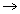bc = a ^^...^^ b , with c up arrows
2. The chain can be reduced in length when the last item is a 1
ab... x1 = ab... x
3. The last item in the chain can be reduced in size by 1
a ... xy( z + 1) depends on y :
1. a ... x1( z + 1) = a ... x
2. a ... x2( z + 1) = a ... x( a ... x )z
3. a ... x3( z + 1) = a ... x( a ... x( a ... x )z )z
4. etc... (where the brackets can be removed once the number inside has been evaluated)

• Ackermann's function becomes even easier to express in right-arrow notation:
•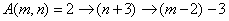• 33642  < Graham's number <  33652  <  3333

Eventually, you get too many right arrows to manipulate easily. Something more extensible is needed...

## Steinhaus's polygon notation

 It is easy to write down very large numbers. Such giants can be defined very simply if we agree to write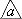instead of a a ,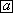instead of ' a in a triangles', and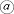instead of ' a in a squares'. Then the number 'Mega' =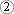is already too great to have any physical meaning,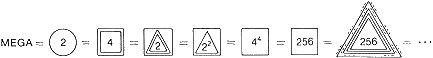the last symbol being 256 in 256 triangles, and the reason why we have abandoned the ordinary system of writing numbers is clear. (The reader may try to explain the 'Megiston' given by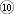). -- H. Steinhaus. Mathematical Snapshots , Ch.1, 3rd edn. 1983 [note that there is an error in the diagram as printed: it should read ...=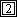= ...]

## Moser's polygon notation

Leo Moser extended Steinhaus' notation. Rather than going to a circle for the third level, Moser continues the sequence of polygons with a pentagon, an hexagon, and so on. So we have

•= a a
•= ' a in a triangles'
•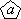= ' a in a squares'
• and so on

In order to express general relationships, it is useful to have a rather different notation, than captures the number of sides of the polygons, and the number of repetitions of the polygons, symbolically. So, instead of drawing the polygon, I write the number of sides in square brackets:= a ;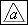= a , etc. And instead of drawing repeated similar polygons, I subscript the 'polygon number' with the repetition number: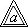= a  = a  2 ;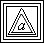= a  = a  2  3 , etc. This means we can define the notation in general:

•= a  = a a
•= a  = ' a in a triangles' = a  a
•= a  = ' a in a squares' = a  a
• a [ k +1] = ' a in a k -gons' = a [ k ] a

The polygons associate to the left, and bind more strongly that conventional mathematical operators. So n ^ n  means ( n )^( n ). Expanding deeply nested terms from the inside out (expand the definition of the innermost polygon first), or from the outside in (expand the definition of the outermost polygon first) gives the same result.

1.= a  = a^a
• n  = n^n
• n  2 = n  = n ^ n  = ( n^n )^( n^n )
• n  3 = n  2  = n  2 ^ n  2 = (( n^n )^( n^n ))^(( n^n )^( n^n ))
• n  4 = n  3  = n  3 ^ n  3 = ((( n^n )^( n^n ))^(( n^n )^( n^n )))^((( n^n )^( n^n ))^(( n^n )^( n^n )))
• n  k = (...( n^n )^( n^n )...)^...^(...( n^n )^( n^n )...)[2 k terms]
• 2 = 2^2 = 4
• 2 2 = 2^2 = 4^4 = 256
• 2 3 = 2 2 ^2 2  = 256^256 = 10 616.509...
• 2 4 = 2 3 ^2 3  = 10 616.509... ^10 616.509... = 10 616.509... x 10 616.509... = 10 10 619.3...
• 2 5 = 2 4 ^2 4 = 10 10 619.3... ^10 10 619.3...
• etc..
• 3 = 3^3 = 27
• 3 2 = 3^3 = 27^27 = 443426488243037769948249630619149892803 = 10 38.646...
• 3 3 = 3 2 ^3 2 = 10 38.646... ^10 38.646... = 10 10 40.23...
• etc...
• 4 = 4^4 = 2^2 = 2 = 2 2
• 4 2 = 4 = 2 2  = 2 3
• 4 3 = 4 2  = 2 3  = 2 4
• 4 k = 2 k +1
2.= a  = a  a
• n  = n  n = (...( n^n )^( n^n )...)^...^(...( n^n )^( n^n )...)[2 n terms]
• 2 = 2 2 = 256
• 2 2 = 2 = 256 = 256 256
• 2 3 = 2 2  = 256 256  = 256 256  256 256
• etc...
• 3 = 3 3 = 3 2 ^3 2 = (27^27)^(27^27)
• 3 2 = 3 3  = (3 2 ^3 2 ) = ((27^27)^(27^27))
= ((27^27)^(27^27)) (27^27)^(27^27)
• etc...
• 4 = 4 4 = 2 5
• etc...
3.= a  = a  a
• 2 = Steinhaus' Mega = 2 2 = 2 2  = (2^2) = (4^4) = 256 256
• A polygon with 2 sides is a Megagon . It looks pretty circular if drawn!
• 2 2 = 2 = 2 2 = 256 256 
• 3 = 3 3 = ((27^27)^(27^27)) (27^27)^(27^27) 
• 10 = Steinhaus' Megiston = 10 10
• etc..
4. a  = a  a
1. 2 = 2 2 = 256 256 
2. 3 = 3 3 = 3 2 = ((27^27)^(27^27)) (27^27)^(27^27)  2
5. in general, a [ k +1] = a [ k ] a

Moser's number = '2 in a Megagon' = 2[2] = 2[256 256 ]

Which of Moser's number and Graham's number is the larger? The Moser construction yields big numbers much faster than does the up-arrow notation, but there are a lot of up-arrows in the Graham number.

• [7 July 1998] : Tim Chow has provided me with a clear outline proof that G >> M .
• Coincidentally, a few days later Todd Cesere, who was the first to suggest to me that "Graham's number is far larger than the Moser", also sent me a proof; it takes a similar approach to Chow's proof.